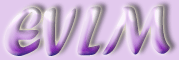Averages

Target: On completion of this worksheet you should understand the differences between the mean, median and the mode and be able to calculate these averages.

An average is a representative value of the data. There are three main types of average: the mean, the median and the mode.

The mode is the most frequent value.

The median is the middle value when the data is arranged in order of magnitude.

The mean is the total of all values divided by the number of values.

# 1  The mode

## 1.1  Examples

1. The number of goals scored by a football team in 9 matches are:

3, 3, 0, 1, 2, 2, 0, 0, 1. Find the mode.

The value which occurs most frequently is 0 so the mode is 0.

2. In the next 8 matches they scored:

4, 3, 1, 3, 0, 4, 4, 3. Find the mode.

In this case both 3 and 4 occur three times so there are two modes 3 and 4. The data is said to be bimodal.

3. The scores for the next 4 matches are: 2, 1, 0, 3. What is the mode?

Each value occurs the same number of times so there is no mode.

# 2  The median

Examples

Find the median for each of the previous examples.

1. First arrange in order:

0, 0, 0, 1, 1, 2, 2, 3, 3. Now find the middle value

0, 0, 0, 1, 1, 2, 2, 3, 3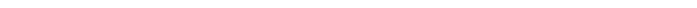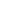notice that there are 9 values and the median is the 5th value (shown in red), in otherwords having value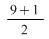.

2. The values arranged in order are:

0, 1, 3, 3, 3, 4, 4, 4. This time there

are two middle values. To find the median add the values and divide by 2. The median is 3. The median falls between the 4th and 5th values or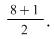3. In order we have: 0, 1, 2, 3. Again there are two middle values, 1 and 2 so the median is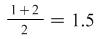. The median is the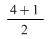= 2.5th value ie between the 2nd and 3rd values.

In general if there are n values then the median is the (n+1)/2 value. For example if there are 11 values then the median is the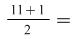6th value. If there are 10 values then the median is the (10+1)/2 = 5.5th value ie between the 5th and 6th values.

# 3  The mean

Mean =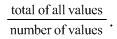## 3.1  Examples

Find the mean for the previous examples:

1. mean =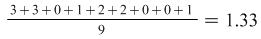2. mean =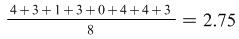3. mean = 2+1+0+3 = 1.5

## 3.2  Examples

1. Find the mean for the following frequency distribution:

 x 2 3 4 5 6 f 3 5 8 4 1

We need to total the x values. There are 3 lots of 2 and 5 lots of 3 etc so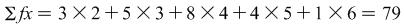(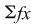is the total of the x values. We say "sigma fx"). There are 21 values altogether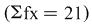mean =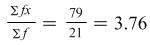2. Find the mean for the grouped frequency distribution:

 class 4-6 6-8 8-10 10-12 f 15 21 12 2

(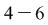means values from 4 to less than 6).

We must use the mid-point of the interval which we find by adding together the class boundaries and dividing by 2. The first one is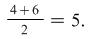We will call these mid-points, x. The method is then the same as in example 1.

 class f x fx 4-6 15 5 75 6-8 21 7 147 8-10 12 9 108 10-12 2 11 22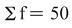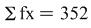Mean =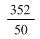= 7·04